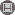Volume 8, Issue 1 (5-2013)                   IJMSI 2013, 8(1): 39-47 | Back to browse issues page

BibTeX | RIS | EndNote | Medlars | ProCite | Reference Manager | RefWorks
Send citation to:Eshaghi Gordji M, Karimipour Samani N, Park C. Approximation of Jordan homomorphisms in Jordan Banach algebras RETRACTED PAPER. IJMSI. 2013; 8 (1) :39-47
URL: http://ijmsi.ir/article-1-401-en.html
Abstract:

In this paper, we investigate the generalized Hyers-Ulam stability of Jordan homomorphisms in Jordan Banach algebras for the functional equation begin{align*} sum_{k=2}^n sum_{i_1=2}^ksum_{i_2=i_{1}+1}^{k+1}cdotssum_{i_n-k+1=i_{n-k}+1}^n fleft(sum_{i=1,i not=i_{1},cdots ,i_{n-k+1}}^n x_{i}-sum_{r=1}^{n-k+1} x_{i_{r}}right) + fleft(sum_{i=1}^{n}x_{i}right)-2^{n-1} f(x_{1}) =0, end{align*} where \$n\$ is an integer greater than 1.

Type of Study: Research paper | Subject: General